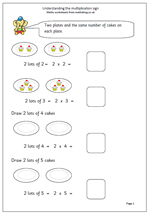# Understanding multiplication in year 1At one time multiplication was not really introduced until year 2, but recent curriculum planning has included understanding multiplication into year 1, so here is a couple of pages to try to help with this.

At first multiplication can be seen as repeated addition so that:

2 added together 3 times is 2 + 2 + 2, or 3 lots of 2, or 3 times 2, or 3 x 2 (or 2 x 3).

The multiplication sign needs to be introduced, including using the words ‘times’, ‘multiply’ and ‘lots of’.

The second of the pages is quite a bit harder as there are no illustrations and various parts of the number sentences are missing. it would be a good idea to ‘talk through’ each number sentence, saying them out loud.

Understand the multiplication sign